# Bernoulli polynomials

(diff) ← Older revision | Latest revision (diff) | Newer revision → (diff)

The polynomials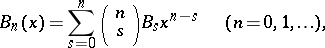where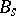are the Bernoulli numbers. Thus, for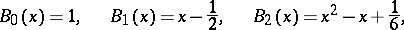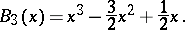Bernoulli polynomials may be computed by the recurrence formula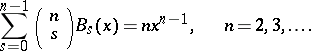For a natural argument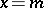Bernoulli polynomials were first studied by Jacob Bernoulli in 1713, in connection with the computation of the sum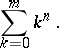L. Euler  was the first to study Bernoulli polynomials for arbitrary values of. The term "Bernoulli polynomials" was introduced by J.L. Raabe in 1851. The fundamental property of such polynomials is that they satisfy the finite-difference equationand therefore play the same role in finite-difference calculus as do power functions in differential calculus.

Bernoulli polynomials belong to the class of Appell polynomials, i.e. they satisfy the conditionand are closely connected with the Euler polynomials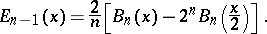The generating function of the Bernoulli polynomials isBernoulli polynomials are expandable into Fourier series: For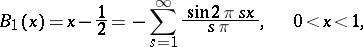and for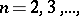Bernoulli polynomials satisfy the relationships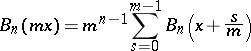(the multiplication theorem);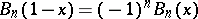(the complement theorem);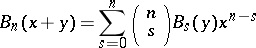(the theorem on addition of arguments).

Bernoulli polynomials are employed to express the residual term of the Euler–MacLaurin formula, and for the expansion of functions into series. Many important properties of Bernoulli numbers are a consequence of the properties of Bernoulli polynomials. Bernoulli polynomials are employed in the integral representation of differentiable periodic functions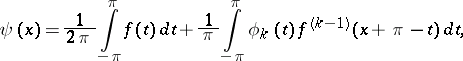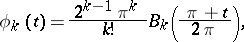and play an important part in the theory of approximation of such functions by trigonometric polynomials and other aggregates. Cf. Favard problem.

Various generalizations of the Bernoulli polynomials have been proposed. N.E. Nörlund introduced generalized Bernoulli polynomials of orderand degree:(certain special cases of such polynomials had been previously considered by V.G. Immenetskii, N.Ya. Sonin and D.M. Sintsov). Letand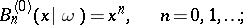thenis successively determined as the polynomial solution of degreeof the finite-difference equation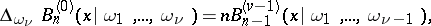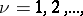with initial conditions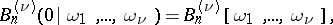where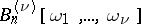(a generalized Bernoulli number) is found from the recurrence relations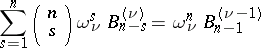How to Cite This Entry:
Bernoulli polynomials. Encyclopedia of Mathematics. URL: http://encyclopediaofmath.org/index.php?title=Bernoulli_polynomials&oldid=19249
This article was adapted from an original article by Yu.N. Subbotin (originator), which appeared in Encyclopedia of Mathematics - ISBN 1402006098. See original article